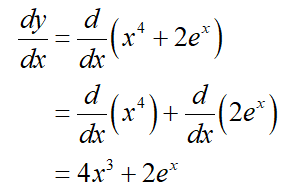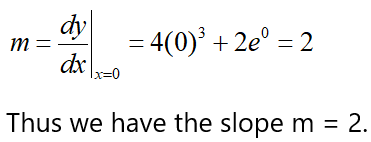# Find the equation of the tangent line to the curve y=x^4+2e^x at the point (0,2).

Question
1 views

Find the equation of the tangent line to the curve y=x^4+2e^x at the point (0,2).

check_circle

Step 1

Consider the given curve

y=x^4+2e^x

Step 2

To find the slope, differentiate the function with respect to x.Step 3

Evaluate the derivative at the given point (0, 2)....

### Want to see the full answer?

See Solution

#### Want to see this answer and more?

Solutions are written by subject experts who are available 24/7. Questions are typically answered within 1 hour.*

See Solution
*Response times may vary by subject and question.
Tagged in